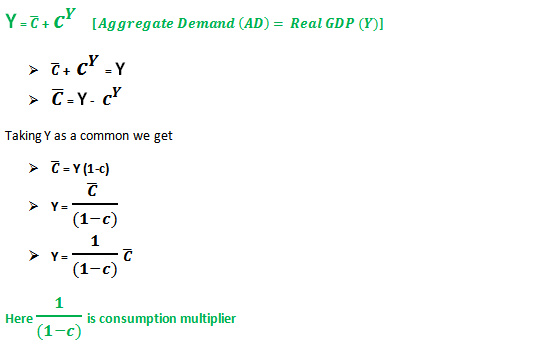## Determination of Consumption MultiplierMarginal Propensity to Consume is the rate of change in consumption for the change in income.In macroeconomics consumption multiplier (CM) is a very old concept but now this is neglected due to more precise National Income Multiplier (NIM). Consumption multiplier helps us to understand other multipliers. It is very simple but it helps us to calculate National Income Multiplier and many other important things in economics.

If autonomous consumption increases when the real gross domestic product (Y) will increase by the amount equal to the increase in autonomous consumption time’s consumption multiplier.

Since MPC is a fraction of small c (0 < c < 1), and consumption multiplier is greater than 1. In other words, if autonomous consumption is increased by \$1000 then Y will increase by more than \$1000. Smaller MPC will produce a small amount of Y and larger MPC will produce a larger amount of Y.

Similarly, a larger value of autonomous consumption will produce the more real gross domestic product (rGDP of Y) and a small value of autonomous consumption will produce a small amount of Y.

Thus it is important to increase both autonomous consumption and marginal propensity to consume.Initially, the equilibrium point is “a” with autonomous consumption of “C bar zero” and real GDP of “Y zero”. If “C bar” increases then the consumption and real GDP will increase, so the curve will shift parallel above the initial line and we get a new equilibrium point “e”. On the other hand if “C bar” decreases then the curve will shift downward parallel to the original line.

Here the change in “Y” is “a to d” but “C” is changed by only “b to e” so it’s clear that (ad > be) “ad” is greater than “be”If there is a change in marginal propensity to consume (small c) then there will be the change in real GDP and the curve will shift, for increase in small c (marginal propensity to consume) curve will shift above the original line but not parallel shift.

Both these cases if there is a change in “C bar” and small “c” real GDP “Y” increase which is good for the economy. So the government should try to increase these.